Miscellaneous

Chapter 11 Class 12 Three Dimensional Geometry
Serial order wise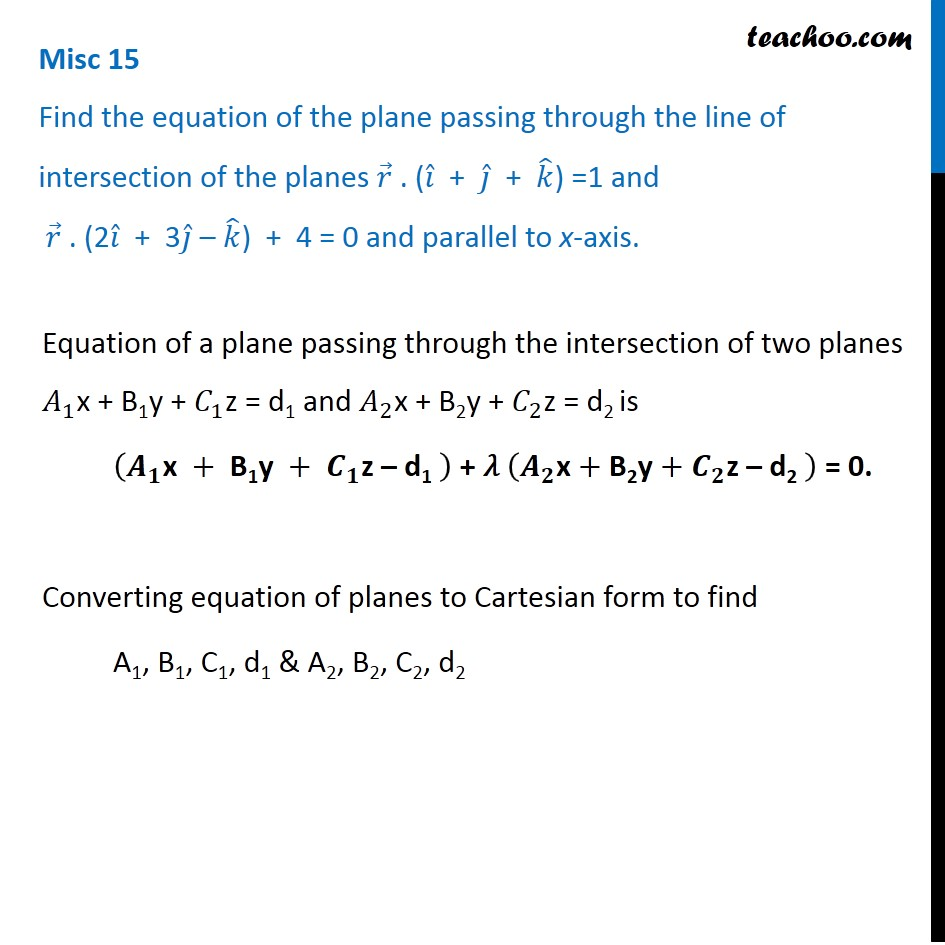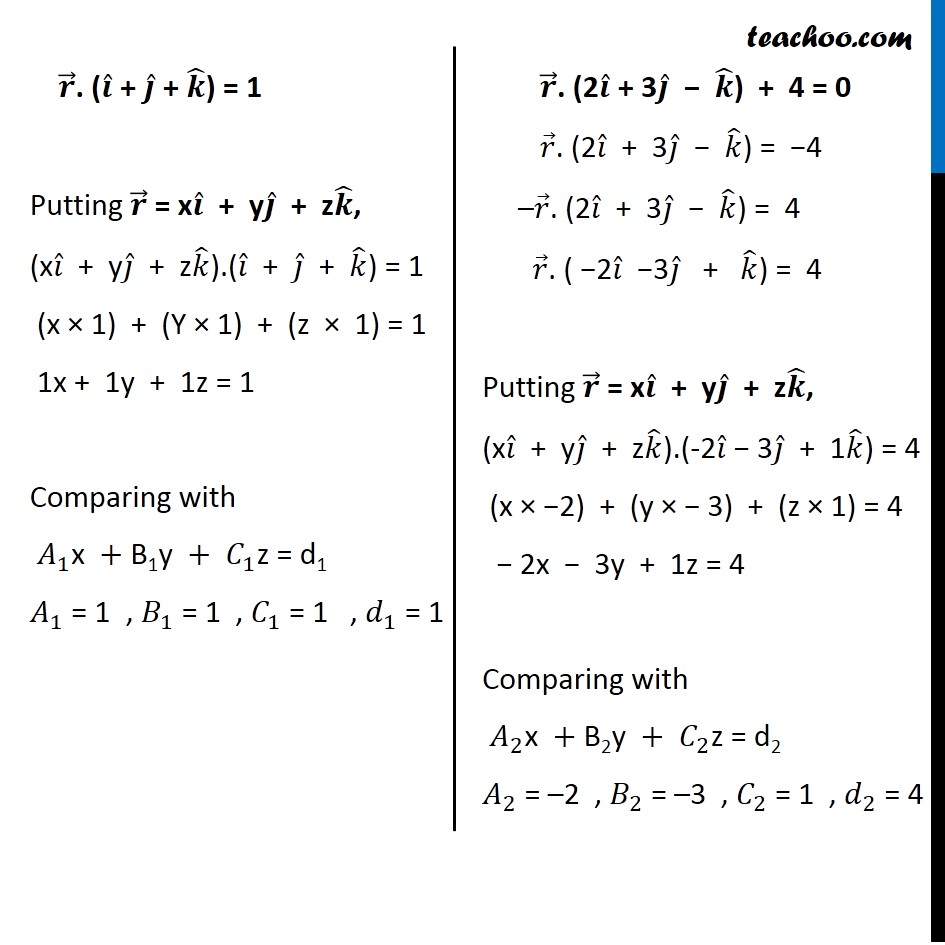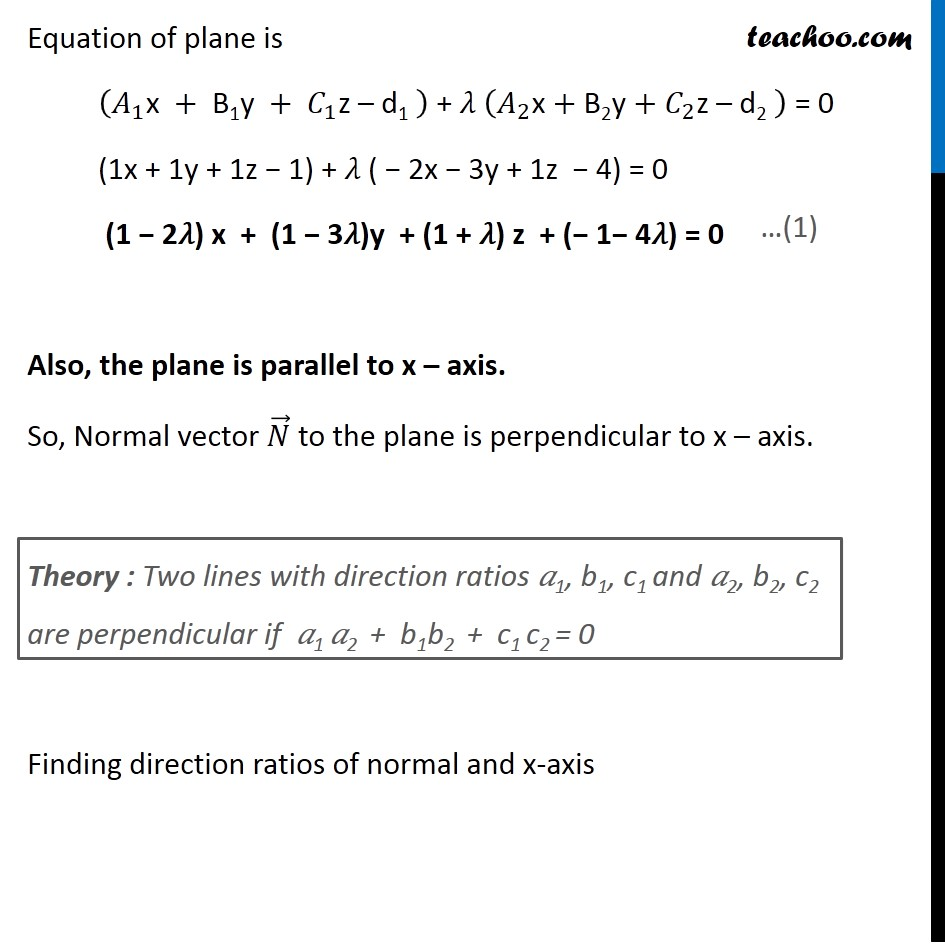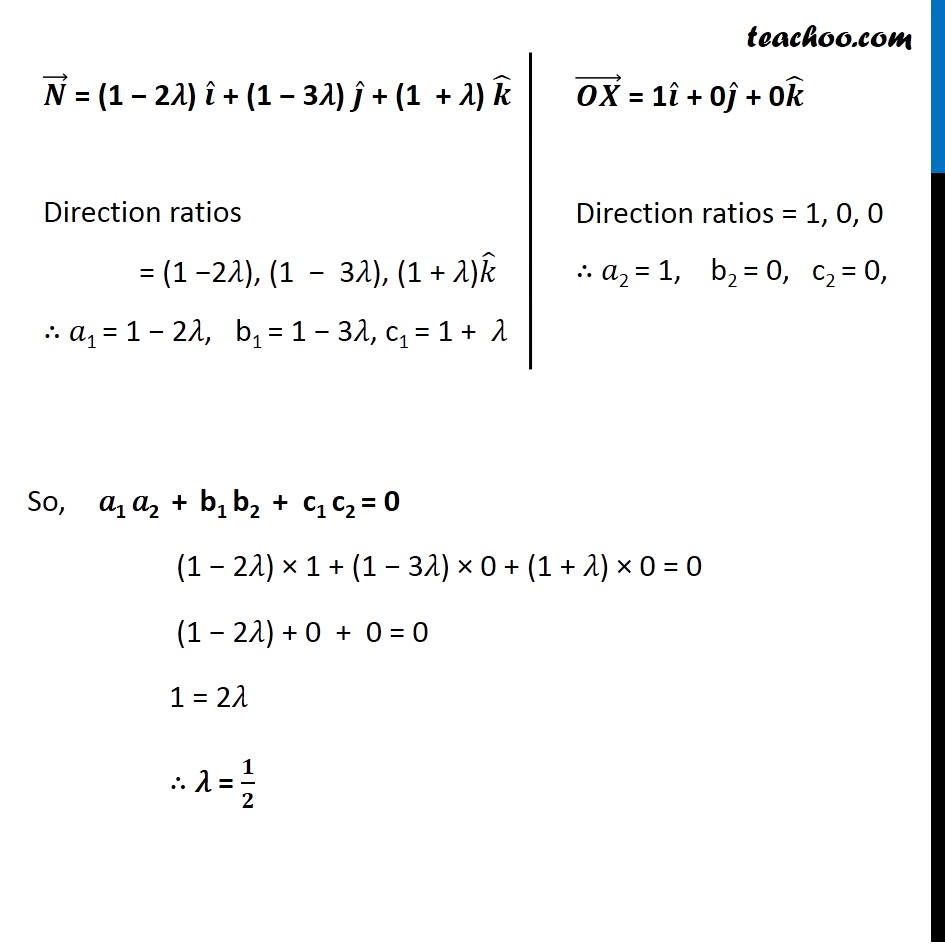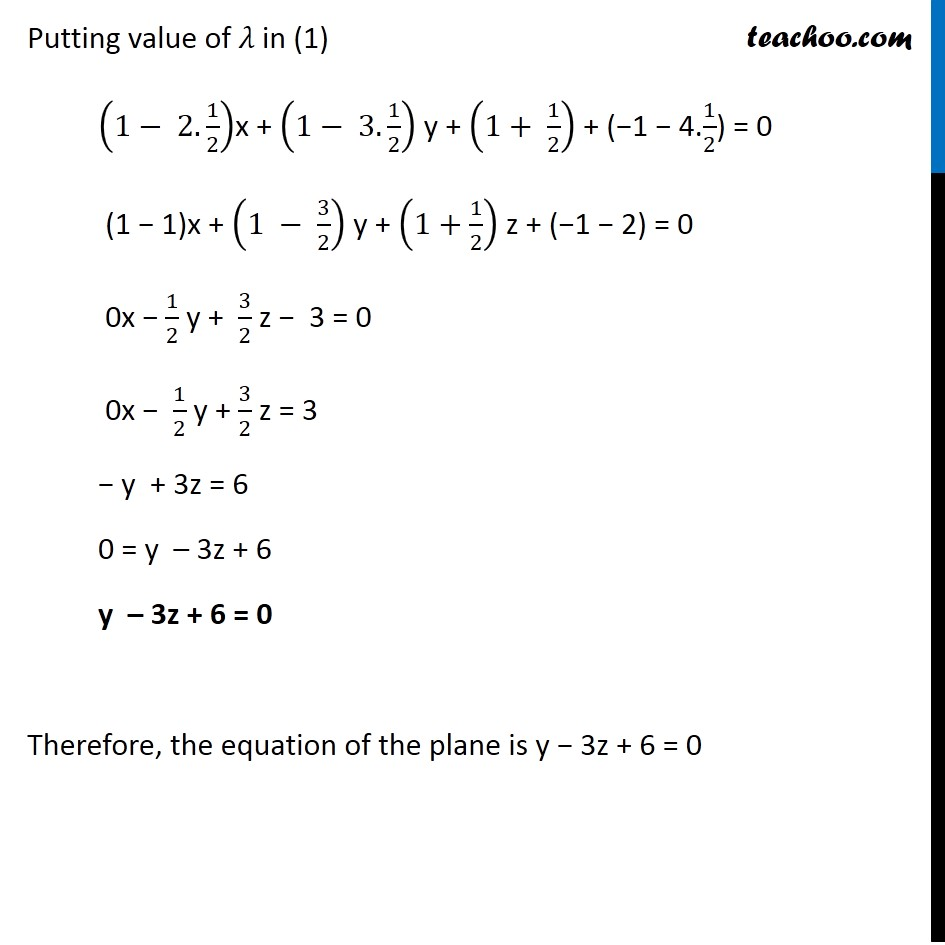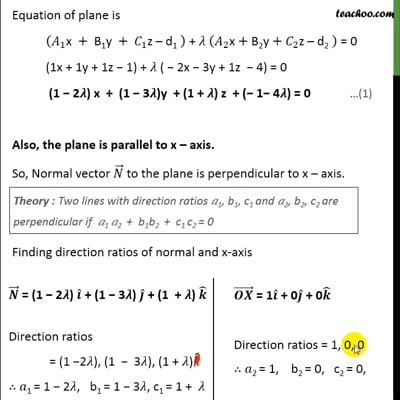This video is only available for Teachoo black users

Get live Maths 1-on-1 Classs - Class 6 to 12

### Transcript

Misc 15 Find the equation of the plane passing through the line of intersection of the planes 𝑟 ⃗ . (𝑖 ̂ + 𝑗 ̂ + 𝑘 ̂) =1 and 𝑟 ⃗ . (2𝑖 ̂ + 3𝑗 ̂ – 𝑘 ̂) + 4 = 0 and parallel to x-axis. Equation of a plane passing through the intersection of two planes 𝐴_1x + B1y + 𝐶_1z = d1 and 𝐴_2x + B2y + 𝐶_2z = d2 is (𝑨_𝟏 "x " +" B1y" + 𝑪_𝟏 "z – d1 " ) + 𝜆 (𝑨_𝟐 "x" +"B2y" +𝑪_𝟐 "z – d2 " ) = 0. Converting equation of planes to Cartesian form to find A1, B1, C1, d1 & A2, B2, C2, d2 𝒓 ⃗. (𝒊 ̂ + 𝒋 ̂ + 𝒌 ̂) = 1 Putting 𝒓 ⃗ = x𝒊 ̂ + y𝒋 ̂ + z𝒌 ̂, (x𝑖 ̂ + y𝑗 ̂ + z𝑘 ̂).(𝑖 ̂ + 𝑗 ̂ + 𝑘 ̂) = 1 (x × 1) + (Y × 1) + (z × 1) = 1 1x + 1y + 1z = 1 Comparing with 𝐴_1 "x "+"B1y "+" " 𝐶_1 "z = d1" 𝐴_1 = 1 , 𝐵_1 = 1 , 𝐶_1 = 1 , 𝑑_1 = 1 𝒓 ⃗. (2𝒊 ̂ + 3𝒋 ̂ − 𝒌 ̂) + 4 = 0 𝑟 ⃗. (2𝑖 ̂ + 3𝑗 ̂ − 𝑘 ̂) = −4 –𝑟 ⃗. (2𝑖 ̂ + 3𝑗 ̂ − 𝑘 ̂) = 4 𝑟 ⃗. ( −2𝑖 ̂ −3𝑗 ̂ + 𝑘 ̂) = 4 Putting 𝒓 ⃗ = x𝒊 ̂ + y𝒋 ̂ + z𝒌 ̂, (x𝑖 ̂ + y𝑗 ̂ + z𝑘 ̂).(-2𝑖 ̂ − 3𝑗 ̂ + 1𝑘 ̂) = 4 (x × −2) + (y × − 3) + (z × 1) = 4 − 2x − 3y + 1z = 4 Comparing with 𝐴_2 "x "+"B2y "+" " 𝐶_2 "z = d2" 𝐴_2 = –2 , 𝐵_2 = –3 , 𝐶_2 = 1 , 𝑑_2 = 4 Equation of plane is (𝐴_1 "x " +" B1y" + 𝐶_1 "z – d1 " ) + 𝜆 (𝐴_2 "x" +"B2y" +𝐶_2 "z – d2 " ) = 0 (1x + 1y + 1z − 1) + 𝜆 ( − 2x − 3y + 1z − 4) = 0 (1 − 2𝜆) x + (1 − 3𝜆)y + (1 + 𝜆) z + (− 1− 4𝜆) = 0 Also, the plane is parallel to x – axis. So, Normal vector 𝑁 ⃗ to the plane is perpendicular to x – axis. Finding direction ratios of normal and x-axis Theory : Two lines with direction ratios 𝑎1, b1, c1 and 𝑎2, b2, c2 are perpendicular if 𝑎1 𝑎2 + b1b2 + c1 c2 = 0 𝑵 ⃗ = (1 − 2𝜆) 𝒊 ̂ + (1 − 3𝜆) 𝒋 ̂ + (1 + 𝜆) 𝒌 ̂ Direction ratios = (1 −2𝜆), (1 − 3𝜆), (1 + 𝜆)𝑘 ̂ ∴ 𝑎1 = 1 − 2𝜆, b1 = 1 − 3𝜆, c1 = 1 + 𝜆 (𝑶𝑿) ⃗ = 1𝒊 ̂ + 0𝒋 ̂ + 0𝒌 ̂ Direction ratios = 1, 0, 0 ∴ 𝑎2 = 1, b2 = 0, c2 = 0, So, 𝑎1 𝑎2 + b1 b2 + c1 c2 = 0 (1 − 2𝜆) × 1 + (1 − 3𝜆) × 0 + (1 + 𝜆) × 0 = 0 (1 − 2𝜆) + 0 + 0 = 0 1 = 2𝜆 ∴ 𝜆 = 𝟏/𝟐 Putting value of 𝜆 in (1) (1− 2. 1/2)x + (1− 3. 1/2) y + (1+ 1/2) + (−1 − 4.1/2) = 0 (1 − 1)x + (1 − 3/2) y + (1+1/2) z + (−1 − 2) = 0 0x − 1/2 y + 3/2 z − 3 = 0 0x − 1/2 y + 3/2 z = 3 − y + 3z = 6 0 = y – 3z + 6 y – 3z + 6 = 0 Therefore, the equation of the plane is y − 3z + 6 = 0